### Home > PC3 > Chapter 8 > Lesson 8.3.5 > Problem8-156

8-156.
1. Let f (x) = x2(xa)2(xb)(xc)3(xd). Assume a < b < 0 < c < d. Homework Help ✎

1. Sketch a possible graph for y = f(x).

2. Solve f(x) ≤ 0.

3. Solve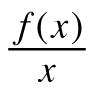≤ 0.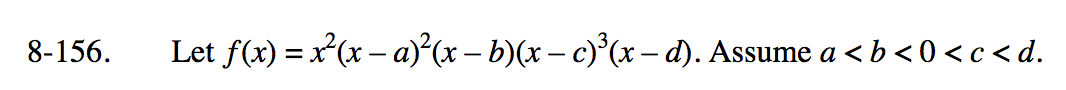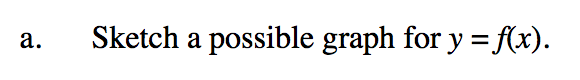Begin by sketching the axes. Mark locations on the x-axis for a, b, c, and d. Then sketch a polynomial curve with roots at x = 0, b, and d, a double root at x = a, and a triple root at x = c.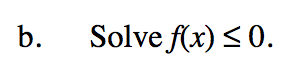Where is your curve below or touching the x-axis?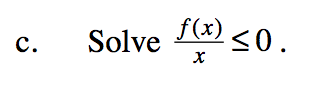When do f(x) and x have opposite signs?
For example, where is the curve above the x-axis on the left side of the x-axis?